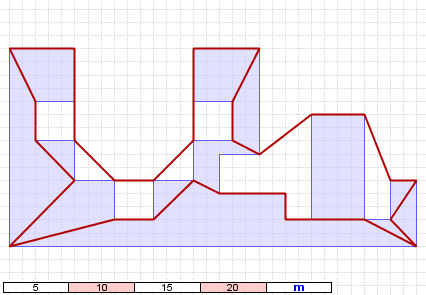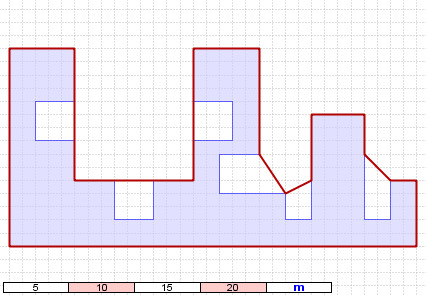# Concave hull based on JTS

### Concave hull

This concave hull implementation is based on the algorithm developed by Duckham et al. (2008) in the paper "Efficient generation of simple polygons for characterizing the shape of a set of points in the plane", available here.

This implementation is based on the JTS Delaunay triangulation, so on the subjacent QuadEdge model. Nevertheless, using directly the QuadEdge model was too complex to obtain optimal performances and a O(n log(n)) algorithm complexity (tests have been done and computational times were quite important -- between 1min30 and 2min to compute the concave hull of a set of 50.000 points). Therefore, the first part of the implementation consists in converting the QuadEdge model into another triangulation model in which relationships between vertices, edges and triangles are clearly defined and easily reachable using accessors.

This implementation enables to consider all types of geometry, i.e. Point, LineString and Polygon, decomposing LineString and Polygon into Point. It is a priori robust (many tests have been done) and a priori efficient -- around or less than 3 seconds to compute "the" concave hull of 50.000 points --. Nevertheless, improvements could still be done (cf. "Some thoughts about concave hull computation" below).

To compute "the" concave hull (a concave hull is not uniquely defined) of a geometry or geometry collection, users have to define a threshold. This threshold is used to remove all the edges which are longer than this threshold during the concave hull creation, except if the edge is part of an irregular triangle (cf. Duckham et al.'s paper for more information).

### Download

The distribution of the concave hull can be downloaded here. This distribution contains four elements:
• concave_hull.jar, binary of the code,
• concave_hull_src.zip, source of the code,
• OJ_concave_hull_plugin.jar, the concave hull OpenJUMP plugin,
• OJ_concave_hull_plugin_src.zip, the source of the OpenJUMP plugin.

### Licences

The concave hull implementation is released under a LGPL licence and the OpenJUMP plugin implementation under GPL, according to JTS and OpenJUMP licences.

### How to use it?

Using through Java code (gc is a GeometryCollection):
ConcaveHull ch = new ConcaveHull(gc, threshold);
Geometry concaveHull = ch.getConcaveHull();

Using through OpenJUMP plugin: Tools menu -> Generate -> Concave hull

### Some thoughts about concave hull computation

Which definition of the threshold? Duckham et al. proposed several solution in their paper to consider an adapted threshold to a set of points.

This implementation could be improved, e.g.:
• when two sets of points are separated by a distance greater to the treshold, a "bridge" is still done between these two sets. It would be better to create a mulitpolygon or several polygons,
• the constrained Delaunay triangulation could be used when the geometries in input are Polygon,
• the merge of the segments of the concave hull which is done by the JTS line merger but it could be done using the vertex ID,
• etc.
Michael Michaud, OpenJUMP developer, tested this implementation (many thanks!) and realised that results were a little bit unexpected when the algorithm is used with layers which contain other geometry than Point, e.g. Polygon as illustrated in Figure 1. Therefore Michael suggests to realise a preprocessing to densify lines with a parameter slightly smaller than the threshold. With such a preprocessing, the concave hull becomes a better representation of the features, as illustrated in Figure 2.Figure 1. Concave hull of a polygon before densification of its exterior ring Figure 2. Concave hull of a polygon after densification of its exterior ring

### For more information

Contact Eric Grosso